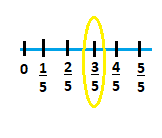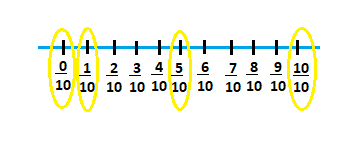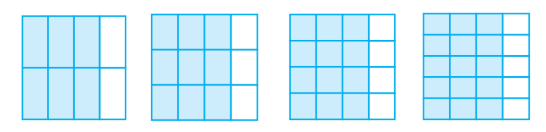# NCERT Solutions for Class 6 Maths Chapter 7 Fractions

NCERT solutions for class 6 maths chapter 7 Fractions- A fraction is a number representing part of a whole. The whole may be a single object or a group of the object and the parts have to be equal. In class 4th and 5th, you have already learnt about the representation of fractions. You already know that one-half is written as 1/2. In this particular chapter, you will learn about the various operations and applications of fractions in mathematics. CBSE NCERT solutions for class 6 maths chapter 7 Fractions will cover the solutions to all these operations and applications of fractions. The subtopics covered under the chapter are the representation of fractions on the number line, proper-improper and mixed fractions, the simplest form of the fractions, equivalent fractions, comparing fractions, comparing, unlike fractions, comparing like fractions, subtraction, and addition of fractions and adding or subtracting fractions. CSE NCERT solutions for class 6 maths chapter 7 Fractions is covering the problems from each subtopic. While expressing a situation of counting parts to write a fraction, you must ensure that all the parts are equal. In p/q, p is called the numerator and q is called the denominator. In this chapter, there are a total of  37 questions in 6 exercises. To help students in their preparation, we have designed solutions of NCERT for class 6 maths chapter 7 Fractions. NCERT Solutions are also available class wise and subject wise. To go to the respective exercise questions click on the link provided below

Exercise 7.1

Exercise 7.2

Exercise 7.3

Exercise 7.4

Exercise 7.5

Exercise 7.6

## NCERT solutions for class 6 maths chapter 7 Fractions Exercise: 7.1(i)

(ii)

(iii)

(iv)

(v)

(vi)

(vii)

(viii)

(ix)

(x)The coloured parts are shown below :-This isThis isThis is

Yes, the above fractions are wrong. For these fractions to be correct areas of each part should be same. But clearly, in the given figure, the areas are not the same.

Total hours in 1 day

Thus the required fraction is :-

We know that 1 hour has 60 minutes.

Thus fraction of 40 minutes is  :-

Arya needs to divide one sandwich into 3 equal parts (as three persons are there.)

Thus each sandwich is divided into 3 parts.

Since they have to distribute 1 part in 3 people, hence the fraction received by each boy will be:-

The required fraction is:-

We have :-  2, 3, 4, 5, 6, 7, 8, 9, 10, 11, 12.

Prime number :-    2, 3, 5, 7, 11.

Thus the fraction of prime numbers is:-

The natural numbers are :-  102, 103, 104, 105, 106, 107, 108, 109, 110, 111, 112, 113.

Prime numbers are :-   103, 107, 109, 113.

Thus fraction is :-The number of boxes with X in them  =  4

Total number of boxes  =  8.

The required fraction is :

CDs and received 5 others as gifts. What fraction of her total

The fraction of the CDs she bought is :

## Topic: Fractions on the Number Line

First we write  as  and  divide the number line into 5 equal parts.First, we write  as  and divide the number line in 10 equal parts.Yes. There are infinite number of fraction between and  (Numerator is less than denominator)

Five more fractions are:

There are infinite number of fractions between  and .

A fraction is of form  and for a number lying between  and , the numerator has to be less than the denominator.

## Solutions for Topic: Proper Fractions

Q1 Give a proper fraction :

(a) whose numerator is  and the denominator is
(b) whose denominator is and the numerator is

(c) whose numerator and denominator add up to . How many fractions of this kind can you make?

(d) whose denominator is more than the numerator.

(Give any five. How many more can you make?)

A proper fraction whose:

(a) the numerator is  and the denominator is  =

(b) denominator is and numerator is   =

(c) numerator and denominator add up to

Pairs of numbers having sum 10 =

Therefore, the proper fractions are

(d) denominator is more than the numerator. =

(a) less than ?

(b) equal to ?

(a) If the numerator is smaller than the denominator, then the fraction will be less than .

(b) If the numerator is equal to the denominator, then the fraction will be equal to .

(a)

(b)

(c)

(d)

(e)

(a)

(b)

(c)

(d)

(e)

## NCERT solutions for class 6 maths chapter 7 Fractions Exercise: 7.2

The number line is given below:-The number line is shown below with the required points marked.The number line locating the given fraction is shown below:-(a)

(b)

(c)

(d)

(e)

(f)

(a)

(b)

(c)

(d)

(e)

(f)

(a)

(b)

(c)

(d)

(e)

(f)

The improper fractions of the mixed fractions are given below :-

(a)

(b)

(c)

(d)

(e)

(f)

## Solutions for NCERT class 6 maths chapter 7 Fractions Topic: Simplest Form of a Fraction

### Q1 Write the simplest form of :

(i)

(ii)

(iii)

(iv)

(v)

(i)

(ii)

(iii)

(iv)

(v)

Yes,  is in its simplest form because 49 and 64 has no common divisor.

## NCERT Solutions for Topic: Equivalent Fractions

and ;  and    are not equivalent because

and

but

Four example of  equivalent fractions are :(i)                                       (ii)                          (iii)                              (iv)

all fractions in it simplest form is

So all fractions are equivalent

## Topic: Equivalent Fractions

(i)

(ii)

(iii)

(iv)

(i)

&nbsnbsp;    (ii)

(iii)

(iv)

## Exercise: 7.3In the case of (a), we have:-

(i)

(ii)

(iii)

(iv)

Hence all fractions are equal in this case.

In the case of (b), we have:-

(i)

(ii)

(iii)

(iv)

(v)The fractions of each are given below :-

(a)

(b)

(c)

(d)

(e)

Similarly,

(i)

(ii)

(iii)

(iv)

(v)

The correct number is 28.

Thus    .

(b)  The correct answer is  16.

The required value is 12.

The required value is 20.

The correct number is   .

Multiplying numerator and denomenator by  .

We have :-

Hence

(a) denominator

(b) numerator

(c) denominator

(d) numerator

(a) Multiply numerator and denomenator by 4, we have :

(b) Multiply numerator and denomenator by 3, we have :

(c) Multiply numerator and denomenator by 6, we have :

(d) Multiply numerator and denomenator by 9, we have :

(a) numerator

(b) denominator

The required equivalent fractions are given below :-

(a)  Divide both numerator and denomenator by 4.

(b) Divide both numerator and denomenator by 12.

Multiply both numerator and denomenator by 6.

Multiply both numerator and denomenator by 4, we get :

Multiply both numerator and denominator by , we get :

Hence these two fractions are not the same.

(a)

(b)

(c)

(d)

(e)

(a)

(b)

(c)

(d)

(e)

The fraction of pencils used by Ramesh is:-

The fraction of pencils used by Sheelu is:-

The fraction of pencils used by Jamaal is:-

Thus, the fractions of pencils used by each are the same.

(i)           (a)

(ii)           (b)

(iii)            (c)

(iv)           (d)

(v)            (e)

(i)

(ii)

(iii)

(iv)

(v)

### Q1 You get one-fifth of a bottle of juice and your sister gets one-third of the same size of a bottle of juice. Who gets more?

My sister gets more because

## NCERT Solutions for Topic: Comparing Like Fractions

### Q1 Which is the larger fraction?

(i)   or

(ii)   or

(iii)   or

The fractions are shown below using greater than or less than sign

(i)

(ii)

(iii)

(a)

(b)

(c)

(a)

(b)

(c)

## Solutions for CBSE class 6 maths chapter 7 Fractions Topic: Arrangement of Fractions

### Q1 (1) Arrange the following in ascending and descending order :

(a)

and

the following in ascending and descending order are  :

(b)

Write 3 more similar examples and arrange them in ascending and descending order.

the following in ascending and descending order are:

(i)

and

(ii)

and

(iii)

and

## NCERT solutions for class 6 maths chapter 7 Fractions Exercise: 7.4(c) Show    on the number line. Put appropriate signs between the fractions given

(a)

(b)

(c)From the above number line we can compare the given numbers easily.

(a)

(b)

(c)

(d)

The comparison is given below  :-

(a)

(b)

(c)

(d)

The five pairs can be :-

,(a)

With the help of given diagram :

Thus(b)

From the diagram it is cleat that :(c)

From the given diagram, we can clearly say that :-(d)

From the diagram it is clear that :-

(a)

(b)

(c)

(d)

(e)

(f)

(g)

(h)

(i)

(j)

(k)

(a)

(b)

(c)

(d)

(e)

(f)

(g)

(h)

(i)

(j)

(k)

(a)

(b)

(c)

(d)

(e)

(f)

(g)

(h)

(i)

( j)

(k)

(l)

(i)

(ii)

(iii)

(iv)

(v)

(vi)

(vii)

(viii)

(ix)

(x)

(xi)

(xii)

Is  equal to

No.

Multiply numerator and denomenator by .

We have :

Hence

Is   equal to   ?

We have .

Is  equal to

Yes.

By multiplying numerator and denominator by 5, we get :

Is   equal to ?

No.

Multiply both numerator and denomenator by 2, we get :-

The fraction of the book read by Ila is:-

So we can compare the fraction now:-

Who exercised for a longer time can be found by comparing the fraction of their work time.

Hence Rohit exercised for a longer time.

In class A, the fraction of students passed with   or above marks :

And, in class B, the fraction is :

Hence the required fraction is same in both the classes.

## Topic: Addition and Subtraction of Fractions

### Q1 My mother divided an apple into equal parts. She gave me two parts and my brother one part. How much apple did she give to both of us together?

mother gave to me  part

mother gave to my brother   part

She gave both off us

part

### Q2 Mother asked Neelu and her brother to pick stones from the wheat. Neelu picked one-fourth of the total stones in it and her brother also picked up one-fourth of the stones. What fraction of the stones did both pick up together?

. Neelu picked stones

brother picked up the stones

The total fraction of the stones they both pick up together

of total stones.

He put covers on Monday=

He put the cover on Tuesday =

and the remaining on Wednesday.

Thus, the fraction of the covers he put on Wednesday =

## Topic: Adding or Substracting like Fractions

### Q1 Find the difference between and .

the difference between and  is given by

## Q2 Mother made a gud patti in a round shape. She divided it into parts. Seema ate one piece from it. If I eat another piece then how much would be left?

Seema ate =

I eat =

Total part eaten is

The left part would be

## Q3 My elder sister divided the watermelon into parts. I ate out of them. My friend ate . How much did we eat between us? How much more of the watermelon did I eat than my friend? What portion of the watermelon remained?

I ate=

My friend ate =

we both eat

the watermelon more I eat than my friend is

The portion of the watermelon remained

## NCERT solutions for class 6 maths chapter 7 Fractions Exercise: 7.5case (b)   - SubtractionQ2 Solve :

(a)

(b)

(c)

(d)

(e)

(f)

(g)

( h)

(i)

(a)

(b)

(c)

(d)

(e)

(f)

(g)

( h)

(i)

Total wall painted   =  Wall painted by Subham + Wall pained by Madhavi

Hence the whole wall is painted by them.

(a)

(b)

(c)

(d)

(a)

or

(b)

or

(c)

(d)

or

The total fraction of oranges in the basket are   .

Thus the fraction of oranges left is :

## Topic: Addition and Subtraction of Fractions

Addition of    and  is given by

Subtraction of   from  is given by

## NCERT solutions for class 6 maths chapter 7 Fractions Exercise: 7.6

Q1 Solve

(a)                    (b)                  (c)

(d)                    (e)                         (f)

(a)

(b)

(c)

(d)

(e)

(f)

Q1 Solve

(g)                         (h)                              (i)

(j)                  (k)                          (l)

(m)                         (n)

(g)

(h)

(i)

(j)

(k)

(l)   \

(m)

(n)

The total length of ribbon is given :-

Thus total length of ribbon is  .

Total amount of cake given to both  =  Cake given to Naina    +    Cake given to Najma

(a)

(b)

(c)

(a)     :-

(b)       :-

(c)      :-The required table is shown below :-The required box is given below :-Let the length of another piece of wire be x.

Thus,

Hence the length of other part is   .

The distance Nandini walked is given by :-

Hence she walked .

If we compare the bookshelves of both, we obtain that :

Also,

Hence the bookshelf of Asha is more full and by  fraction.

Firstly, let us convert the time taken by Jaidev in improper fraction from mixed fraction.

Now, comparing both, we have :

Also,

Hence Rahul takes less time as compared to Jaidev by      a fraction.

## NCERT solutions for class 6 mathematics chapter-wise

 Chapters No. Chapters Name Chapter - 1 NCERT solutions for class 6 maths chapter 1 Knowing Our Numbers Chapter - 2 Solutions of NCERT for class 6 maths chapter 2 Whole Numbers Chapter - 3 CBSE NCERT solutions for class 6 maths chapter 3 Playing with Numbers Chapter - 4 NCERT solutions for class 6 maths chapter 4 Basic Geometrical Ideas Chapter - 5 Solutions of NCERT for class 6 maths chapter 5 Understanding Elementary Shapes Chapter - 6 CBSE NCERT solutions for class 6 maths chapter 6 Integers Chapter - 7 NCERT solutions for class 6 maths chapter 7 Fractions Chapter - 8 Solutions of NCERT for class 6 maths chapter 8 Decimals Chapter - 9 CBSE NCERT solutions for class 6 maths chapter 9 Data Handling Chapter -10 NCERT solutions for class 6 maths chapter 10 Mensuration Chapter -11 Solutions of NCERT for class 6 maths chapter 11 Algebra Chapter -12 CBSE NCERT solutions for class 6 maths chapter 12 Ratio and Proportion Chapter -13 NCERT solutions for class 6 maths chapter 13 Symmetry Chapter -14 Solutions of NCERT for class 6 maths chapter 14 Practical Geometry

## NCERT solutions for class 6 subject wise

 NCERT Solutions for class 6 maths Solutions of NCERT for class 6 science

## How to use NCERT solutions for class 6 maths chapter 7 Fractions?

• Learn the representation of fractions using the equal part concept.
• Go through the text given in the textbook to learn various applications and concepts.
• Have a glance through some examples to understand the answering of a particular kind of question.
• While practicing you can use NCERT solutions for class 6 maths chapter 7 Fractions as a helping tool.

Keep learning and working hard!